# Basic principles of strain gauge technology

Basic information on the technological field of "strain gauges/load cells" as metrological instruments is to be given below. The information is of general nature; it is up to the user to check the extent to which it applies to his application.

Strain gauges serve either to directly measure the static (0 to a few Hz) or dynamic (up to several KHz) elongations, compressions or torsions of a body by being directly fixed to it, or to measure various forces or movements as part of a sensor (e.g. load cells/force transducers, displacement sensor, vibration sensors).
In the case of the optical strain gauge (e.g. Bragg grating), an application of force causes a proportional change in the optical characteristics of a fiber used as a sensor. Light with a certain wavelength is fed into the sensor. Depending upon the deformation of the grating, which is laser-cut into the sensor, due to the mechanical load, part of the light is reflected and evaluated using a suitable measuring transducer (interrogator).

The commonest principle in the industrial environment is the electrical strain gauge. There are many common terms for this type of sensor: load cell, weighbridge, etc.

## Structure of electrical strain gauges

A strain gauge consists of a carrier material (e.g. stretchable plastic film) with an applied metal film from which a lattice of electrically conductive resistive material is worked in very different geometrical forms, depending on the requirements.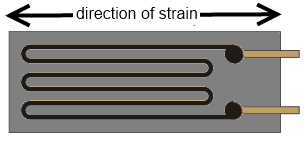Strain gauge

This utilizes a behavior whereby, for example in the case of strain, the length of a metallic resistance network increases and its diameter decreases, as a result of which its electrical resistance increases proportionally.

ΔR/R = k*ε

ε = Δl/l thereby corresponds to the elongation; the strain sensitivity is called the k-factor. This also gives rise to the typical track layout inside the strain gauge: the resistor track or course is laid in a meandering pattern in order to expose the longest possible length to the strain.

Example

The elongation ε = 0.1 % of a strain gauge with k-factor 2 causes an increase in the resistance of 0.2 %. Typical resistive materials are constantan (k~2) or platinum tungsten (92PT, 8W with k ~4). In the case of semiconductor strain gauges a silicon structure is glued to a carrier material. The conductivity is changed primarily by deformation of the crystal lattice (piezo-resistive effect); k-factors of up to 200 can be achieved.

## Measurement of signals

The change in resistance of an individual strain gauge can be determined in principle by resistance measurement (current/voltage measurement) using a 2/3/4-conductor measurement technique

Usually 1/2/4 strain gauges are arranged in a Wheatstone bridge (-> quarter/half/full bridge); the nominal resistance/impedance R0 of all strain gauges (and the auxiliary resistors used if necessary) is usually equivalent to R1=R2=R3=R4=R0. Typical values in the non-loaded state are R0 = 120 Ω, 350 Ω, 700 Ω and 1 kΩ.

The full bridge possesses the best characteristics such as linearity in the feeding of current/voltage, four times the sensitivity of the quarter-bridge as well as systematic compensation of disturbing influences such as temperature drift and creeping. In order to achieve high sensitivity, the 4 individual strain gauges are arranged on the carrier in such a way that 2 are elongated and 2 are compressed in each case.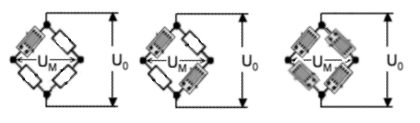quarter, half and full bridge

The measuring bridges can be operated with constant current, constant voltage, or also with AC voltage using the carrier frequency method.Measuring procedureThe Beckhoff EL/KL335x Terminals and the EP3356 Box support only the constant excitation
Full bridge strain gauge at constant voltage (ratiometric measurement)

Since the relative resistance change ΔR is low in relation to the nominal resistance R0, a simplified equation is given for the strain gauge in the Wheatstone bridge arrangement:

UD/UV = ¼ * (ΔR1-ΔR2+ΔR3-ΔR4)/R0

A suitable measuring instrument measures the bridge supply voltage UV (or USupply) and the resulting bridge voltage UD (or UBridge), and forms the quotients from both voltages, i.e. the ratio. After further calculation and scaling the measured value is output, e.g. in kg. Due to the division of UD and UV the measurement is in principle independent of changes in the supply voltage.

If the voltages UV and UD are measured simultaneously, i.e. at the same moment, and placed in relation to each other, then this is referred to as a ratiometric measurement.

The advantage of this is that (with simultaneous measurement!) brief changes in the supply voltage (e.g. EMC effects) or a generally inaccurate or unstable supply voltage likewise have no effect on the measurement.

A change in UV by e.g. 1 % creates the same percentage change in UD according to the above equation. Due to the simultaneous measurement of UD and UV the error cancels itself out completely during the division.

## 4-conductor vs. 6-conductor connection

If supplied with a constant voltage of 5 to 12 V a not insignificant current flows of e.g. 12 V/350 Ω = 34.3 mA. This leads not only to dissipated heat, wherein the specification of the strain gauge employed must not be exceeded, but possibly also to measuring errors in the case of inadequate wiring due to line losses not being taken into account or compensated.

In principle a full bridge can be operated with a 4-conductor connection (2 conductors for the supply UV and 2 for the measurement of the bridge voltage UD).

If, for example, a 25 m copper cable (feed + return = 50 m) with a cross section q of 0.25 mm² is used, this results in a line resistance of

RL = l/ (κ * q) = 50 m / (58 S*m/mm² * 0.25 mm²) = 3.5 Ω

If this value remains constant, then the error resulting from it can be calibrated out. However, assuming a realistic temperature change of, for example, 30° the line resistance RL changes by

ΔRL =30° * 3.9 * 10-4 * 3.5 Ω = 0.41 Ω

In relation to a 350 Ω measuring bridge this means a measuring error of > 0.1 %.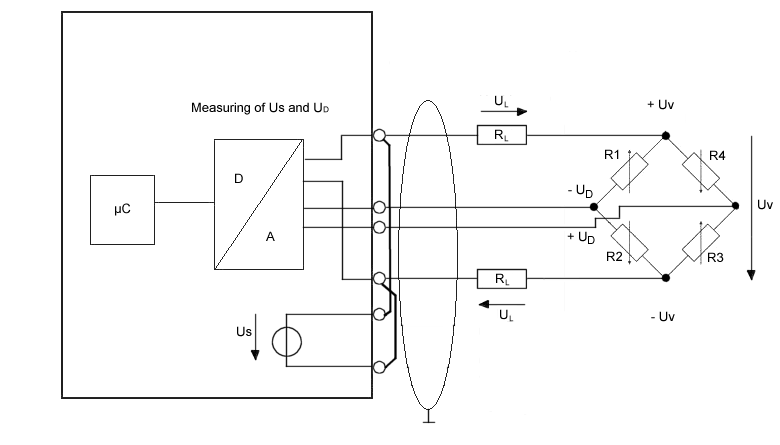4-conductor connection

This can be remedied by a 6-conductor connection, in particular for precision applications (only possible with EL3356).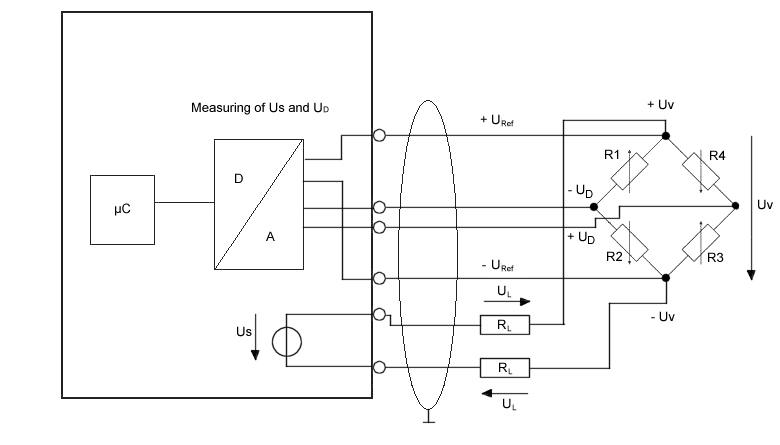6-conductor connection

The supply voltage UV is thereby fed to the strain gauge (= current carrying conductor). The incoming supply voltage Uref is only measured with high impedance directly at the measuring bridge in exactly the same way as the bridge voltage UD with two currentless return conductors in each case. The conductor-related errors are hence omitted.

Since these are very small voltage levels of the order of mV and µV, all conductors should be shielded. The shield must be connected to pin 5 of the M12 connector.EP3356-0022: No 6-conductor connection necessaryThe connection of a strain gauge over 4-conductor with the EP3356-0022 is sufficient because due to the shorter cable lengths no measurement errors occur.

## Structure of a load cell with a strain gauge

One application of the strain gauge is the construction of load cells.

This involves gluing strain gauges (full bridges as a rule) to an elastic mechanical carrier, e.g. a double-bending beam spring element, and additionally covered to protect against environmental influences.

The individual strain gauges are aligned for maximum output signals according to the load direction (2 strain gauges in the elongation direction and 2 in the compression direction).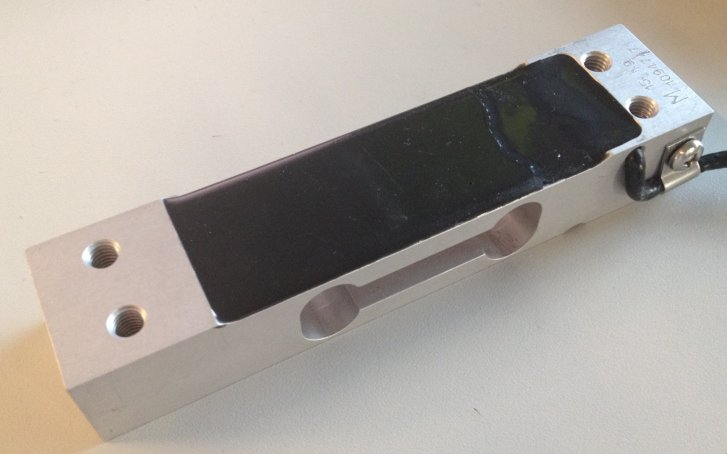## The most important characteristic data of a load cellCharacteristic dataPlease enquire tot he sensor manufacturer regarding the exact characteristic data!

Maximum permissible load for normal operation, e.g. 10 kg

Nominal characteristic value mV/V

The nominal characteristic value 2 mV/V means that, with a supply of US=10 V and at the full load Emax of the load cell, the maximum output voltage UD = 10 V * 2 mV/V *E = 20 mV. The nominal characteristic value is always a nominal value – a manufacturer’s test report is included with good load cells stating the characteristic value determined for the individual load cell, e.g. 2.0782 mV/V.

## Minimum calibration value Vmin

This indicates the smallest mass that can be measured without the maximum permissible error of the load cell being exceeded [RevT].

This value is represented either by the equation Vmin = Emax / n (where n is an integer, e.g. 10000), or in % of Emax (e.g. 0.01).

This means that a load cell with Emax = 10 kg has a maximum resolution of

Vmin = 10 kg / 10000 = 1 g or Vmin = 10 kg * 0.01 % = 1 g.

## Accuracy class according to OIML R60

The accuracy class is indicated by a letter (A, B, C or D) and an additional number, which encodes the scale interval d with a maximum number nmax (*1000); e.g. C4 means Class C with maximally 4000d scale intervals.

The classes specify a maximum and minimum limit for scale intervals d:

A: 50,000 – unlimited
B: 5000 – 100,000
C: 500 – 10,000
D: 500 – 1000

The scale interval nmax = 4000d states that, with a load cell with a resolution of Vmin = 1 g, a calibratable set of scales can be built that has a maximum measuring range of 4000d * Vmin = 4 kg. Since Vmin is thereby a minimum specification, an 8 kg set of scales could be built – if the application allows – with the same load cell, wherein the calibratable resolution would then fall to 8 kg/4000d = 2 g. From another point of view the scale interval nmax is a maximum specification; hence, the above load cell could be used to build a set of scales with a measuring range of 4 kg, but a resolution of only 2000 divisions = 2 g, if this is adequate for the respective application. Also the classes differ in certain error limits related to non-repeatability/creep/TC

## Accuracy class according to PTB

The European accuracy classes are defined in an almost identical way (source: PTB).

Class
Calibration values
Max/e

Minimum value
Maximum value
|
Fine scales
0.001 g <= e
100 e
50000

||
Precision scales
g <= e <=0.05 g
g <= e
20 e
50 e
100
5000
100000
100000
|||
Commercial scales
g <= e <=2 g
g <= e
20 e
20 e
100
500
10000
10000
||||
Coarse scales
5 g <= e
10 e
100
1000

## Minimum application range or minimum measuring range in % of rated load

This is the minimum measuring range/measuring range interval, which a calibratable load cell/set of scales must cover.

Example: above load cell Emax = 10 kg; minimum application range e.g. 40 % Emax

The used measuring range of the load cell must be at least 4 kg. The minimum application range can lie in any range between Emin and Emax, e.g. between 2 kg and 6 kg if a tare mass of 2 kg already exists for structural reasons. A relationship between nmax and Vmin is thereby likewise apparent: 4000 * 1 g = 4 kg .

There are further important characteristic values, which are for the most part self-explanatory and need not be discussed further here, such as nominal characteristic value tolerance, input/output resistance, recommended supply voltage, nominal temperature range etc.

## Parallel connection of strain gauges

It is usual to distribute a load mechanically to several strain gauge load cells at the same time. Hence, for example, the 3-point bearing of a silo container on 3 load cells can be realized. Taking into account wind loads and loading dynamics, the total loading of the silo including the dead weight of the container can thus be measured.

The load cells connected mechanically in parallel are usually also connected electrically in parallel.
Since the four M12 sockets of the EP3356 are internally connected to one another, an external parallel connection is not necessary: if several load cells are connected to the EP3356, they are automatically connected in parallel. Up to four load cells can be connected.

The three load cells in the above example (silo container) can thus be connected to any three of the M12 sockets of the EP3356.

Note:

the load cells must be matched to each other and approved by the manufacturer for this mode of operation
the impedance of the load cells must be large enough that the maximum output current of the reference voltage Uref is not exceeded: 350 mA.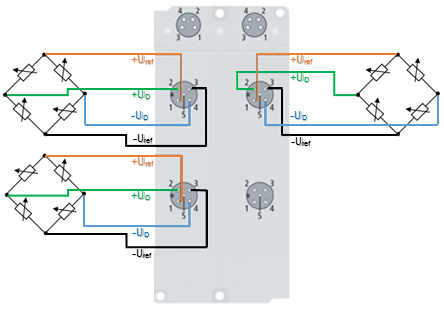Example: Parallel connection of three load cells. Up to four load cells can be connected in parallel.

## Sources of error/disturbance variables

Inherent electrical noise of the load cell

Electrical conductors exhibit so-called thermal noise (thermal/Johnson noise), which is caused by irregular temperature-dependent movements of the electrons in the conductor material. The resolution of the bridge signal is already limited by this physical effect. The rms value en of the noise can be calculated by en = √4kTRB

In the case of a load cell with R0 = 350 Ω at an ambient temperature T = 20 °C (= 293 K) and a bandwidth of the measuring transducer of 50 Hz (and Boltzmann constant k = 1.38 * 10-23 J/K), the rms en= 16.8 nV. The peak-peak noise epp is thus approx. epp ~ 4* en = 67.3 nV.

Example:

In relation to the maximum output voltage Uout-max of a bridge with 2 mV/V and Us = 5 V, this corresponds to Uout-max = 5 V * 2 mV/V = 10 mV. (For the nominal load) this results in a maximum resolution of 10 mV/67.3 nV = 148588 digits. Converted into bit resolution: ln(148588)/ln(2) = 17 bits. Interpretation: a higher digital measuring resolution than 17 bits is thus inappropriate for such an analog signal in the first step. If a higher measuring resolution is used, then additional measures may need to be taken in the evaluation chain in order to obtain the higher information content from the signal, e.g. hardware low-pass filter or software algorithms.

This resolution applies alone to the measuring bridge without any further interferences. The resolution of the measuring signal can be increased by reducing the bandwidth of the measuring unit.

If the strain gauge is glued to a carrier (load cell) and wired up, both external electrical disturbances (e.g. thermovoltage at connection points) and mechanical vibrations in the vicinity (machines, drives, transformers (mechanical and audible 50 Hz vibration due to magnetostriction etc.)) can additionally impair the result of measurement.

Creep

Under a constant load, spring materials can further deform in the load direction. This process is reversible, but it generates a slowly changing measured value during the static measurement. In an ideal case the error can be compensated by constructive measures (geometry, adhesives).

Hysteresis

If even elongation and compression of the load cell take place, then the output voltage does not follow exactly the same curve, since the deformation of the strain gauge and the carrier may be different due to the adhesive and its layer thickness.

## Temperature drift (inherent heating, ambient temperature)

Relatively large currents can flow in strain gauge applications, e.g. I = US/R0 = 10 V / 350 Ω = 26 mA. The power dissipation at the sensor is thus P= U*I = 10 V * 26 mA = 260 mW. Depending on application/carrier material (= cooling) and ambient temperature, a not insignificant error can arise that is termed apparent elongation. The sensor manufacturers integrate suitable compensation elements in their strain gauges.